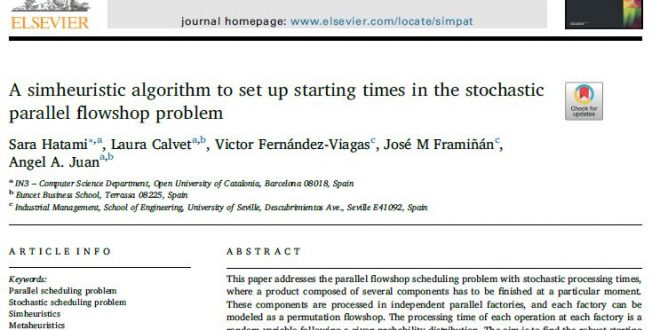آخرین خبرها# الگوریتم Simureistic برای تنظیم زمان شروع در مسئله جریان موازی تصادفی

This paper addresses the parallel flowshop scheduling problem with stochastic processing times, where a product composed of several components has to be finished at a particular moment. These components are processed in independent parallel factories, and each factory can be modeled as a permutation flowshop. The processing time of each operation at each factory is a random variable following a given probability distribution. The aim is to find the robust starting time of the operations at each factory in a way that all the components of the product are completed on a given deadline with a user-defined probability. A simheuristic algorithm is proposed in order to minimize each of the following key performance indicators: (i) the makespan in the deterministic version; and (ii) the expected makespan or a makespan percentile in the stochastic version. A set of computational experiments are carried out to illustrate the performance of the proposed methodology by comparing the outputs under different levels of stochasticity.

نویسندگان: Sara Hatami, Laura Calvet, Victor Fernández-Viagas, José M Framiñán, Angel A.Juan

ژورنال: Simulation Modelling Practice and Theory

سال انتشار: 2018

دانلود مقاله: